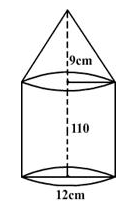QUESTION

# A solid iron pole having cylindrical portion 110 cm high and of base diameter 12 cm is surmounted by a cone 9 cm high. Find the mass of the pole, given that the mass of $1\,c{m^3}$ of iron is 8 gm.

Hint: For solving this type of questions, we will find the volume of cylinder and cone separately and then add them to find the volume of the pole. Then we will convert the volume of the pole into mass of the pole with the help of given condition and solve accordingly.

Complete step-by-step solution -
All the measurements in the figure below are in cm.Base diameter of the cylindrical portion = d,
d = 12 cm
Base radius of the cylindrical portion = r,
$r = \dfrac{d}{2}$ ,
r = 6 cm,
Base radius of the cylindrical portion = Base radius of cone. Therefore,
Base radius of cone, r = 6 cm,
Height of the cylinder, H = 110 cm
Height of the cone, h = 9 cm
Volume of cylinder = $\pi {r^2}H$
Volume of cone = $\dfrac{1}{3}\pi {r^2}h$
Volume of the pole, V = Volume of the cylinder + Volume of the cone

$\Rightarrow V = \pi {r^2}\left( {H + \dfrac{1}{3}h} \right)$
Now substituting the values of r, h and H, we get,
$\Rightarrow V = \pi {6^2}\left( {110 + \dfrac{1}{3} \times {\text{ }}9} \right)$
$\Rightarrow V{\text{ }} = {\text{ }}36\pi \left( {110{\text{ }} + {\text{ }}3} \right)$
$\Rightarrow V = 36 \times \dfrac{{22}}{7} \times 113$
$\Rightarrow V = \dfrac{{89496}}{7}$
Volume of the pole,
$\Rightarrow V = 12,785.14{\text{ c}}{{\text{m}}^3}$
Given mass of 1 cm³ of the iron pole = 8 gm,
Mass of 12785.14 cm³ of the iron pole,
$\Rightarrow M = \;8 \times 12785.14$
$\begin{array}{*{20}{l}} { \Rightarrow M = {\text{ }}102281.12{\text{ }}gm} \\ { \Rightarrow M = {\text{ }}\dfrac{{102281.12}}{{1000}}{\text{ }}kg\,\,\,\,\,\,\,\,\,\,\,\,\,[1g = \dfrac{1}{{1000}}kg]} \end{array}$
$\Rightarrow M = 102.3kg$
Mass of the iron pole,
M = 102.3 kg.
Hence, the mass of the pole = 102.3 kg.

Note: The basic problem faced in this type of questions is calculation problem. While solving this problem we must take care of units and convert units into suitable form whenever required. While taking the value of $\pi$ there is a chance of calculation error which can be avoided by carefully substituting the values.